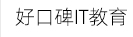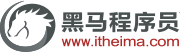### 全国中心# Java异常之throws关键字用法介绍

throws关键字声明抛出异常的语法格式如下:```public class Example1{
public static void main(String [] args){
int result=divide(4,2);     //调用 divide()方法
System.out.println(result);  //下面的方法实现了两个整数相除，并使用 throws关键字声明抛出异常
}
public static int divide(int x ,int y) throws Exception{
int result=x / y;  //定义一个变量 result记录两个数相除的结果
return result;  //将结果返回
}
}```

D:\cn\itcast\chapter04>java Example1.java

Example1.java:3: 错误：未报告的异常错误Exception；必须对其进行捕获或者声明以便抛出

int result divide(4, 2)；//调用 divide()方法

1个错误

```public class Example2{
public static void main(String [] args){
try {
int result= divide(4,2);    //调用 divide()方法
System.out.println(result);
} catch( Exception e) {   //对捕获到的异常进行处理
e.printstackTrace(); //打印捕获的异常信息
}
}
//下面的方法实现了两个整数相除，并使用 throws关键字声明抛出异常
public static int divide (int x, int y) throws Exception {
int result=x / y;   //定义一个变量resu1t记录两个数相除的结果
return result;
}
}```

D:\cn\itcast\chapter04>java Example2

2

```public class Example3{
public static void main(String[] args) throws Exception {
int result= divide(4，0); //调用divide()方法
System.out.println(result);
}
//下面的方法实现了两个整数相除，并使用throws关键词字声明抛出异常
public static int divide(int x,int y) throws Exception {
int result=x /y;  //定义一个变量result记录两个数相除的结果
return result;  //将结果返回
}
}```

D:\cn\itcast\chapter04>java Example3

Exception in thread “main” java.lang.ArithmeticException:/  by zero

at Example25.divide(Exaple3.java:8)

at exmaple25.main(Examle3.java3)